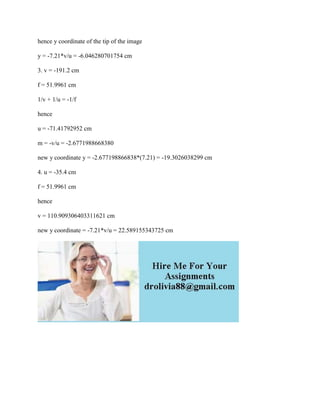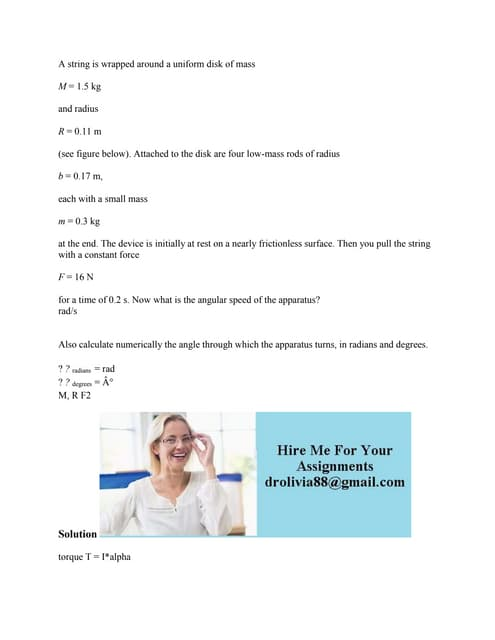Anúncio

# A spherical mirror forms an image of an arrow atx x2956 om- The mirror.docx

3 de Feb de 2023Próximos SlideSharesA string is wrapped around a uniform disk of mass M - 1-5 kg and radiu.docx
Carregando em ... 3
1 de 2
Anúncio

### A spherical mirror forms an image of an arrow atx x2956 om- The mirror.docx

1. A spherical mirror forms an image of an arrow atx x2956 om. The mirror is represented in the drawing as a plane atx -0. The tip of the object arrow is located at(xy) (x1y) (-114cm, 7.21 cm). 4y (xy) ") What is f, the focal length of the mirror? If the mirror is concave f is positive If the mirror is conve f is negative Subm 2) What is y2, the y co-ordinate of the image of the tip of the arrow? 3) The object arrow is now moved such that image distance doubles, i e, Ximage.new - 191 2 cm. What is yimagenew. the new y co-ordinate of the image of the tip of the arrow? 4) The object arrow is now moved to x -x1new 35.4 cm. What is y2new. the new y co-ordinate of the mage of the arrow? cm Submit Solution given spherical mirro let focal length be f then for concave mirror the focal length is +ve ( given) 1. object position, u = -114 cm v = -95.6 cm mirror equation 1/v + 1/u = -1/f -1/95.6 - 1/114 = -1/f hence f = 51.99618320 cm hence the mirror is concave 2. magnification = -v/u = 0.838596491228
2. hence y coordinate of the tip of the image y = -7.21*v/u = -6.046280701754 cm 3. v = -191.2 cm f = 51.9961 cm 1/v + 1/u = -1/f hence u = -71.41792952 cm m = -v/u = -2.6771988668380 new y coordinate y = -2.677198866838*(7.21) = -19.3026038299 cm 4. u = -35.4 cm f = 51.9961 cm hence v = 110.909306403311621 cm new y coordinate = -7.21*v/u = 22.589155343725 cm
Anúncio